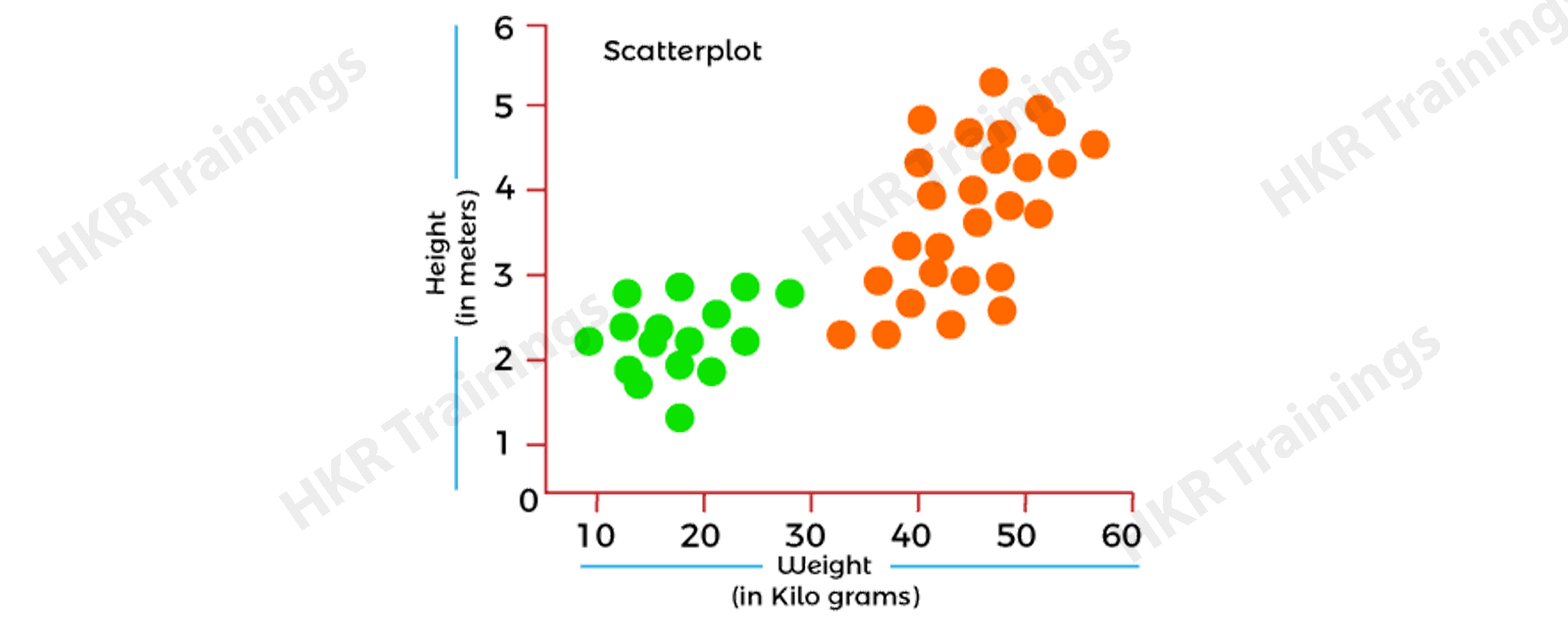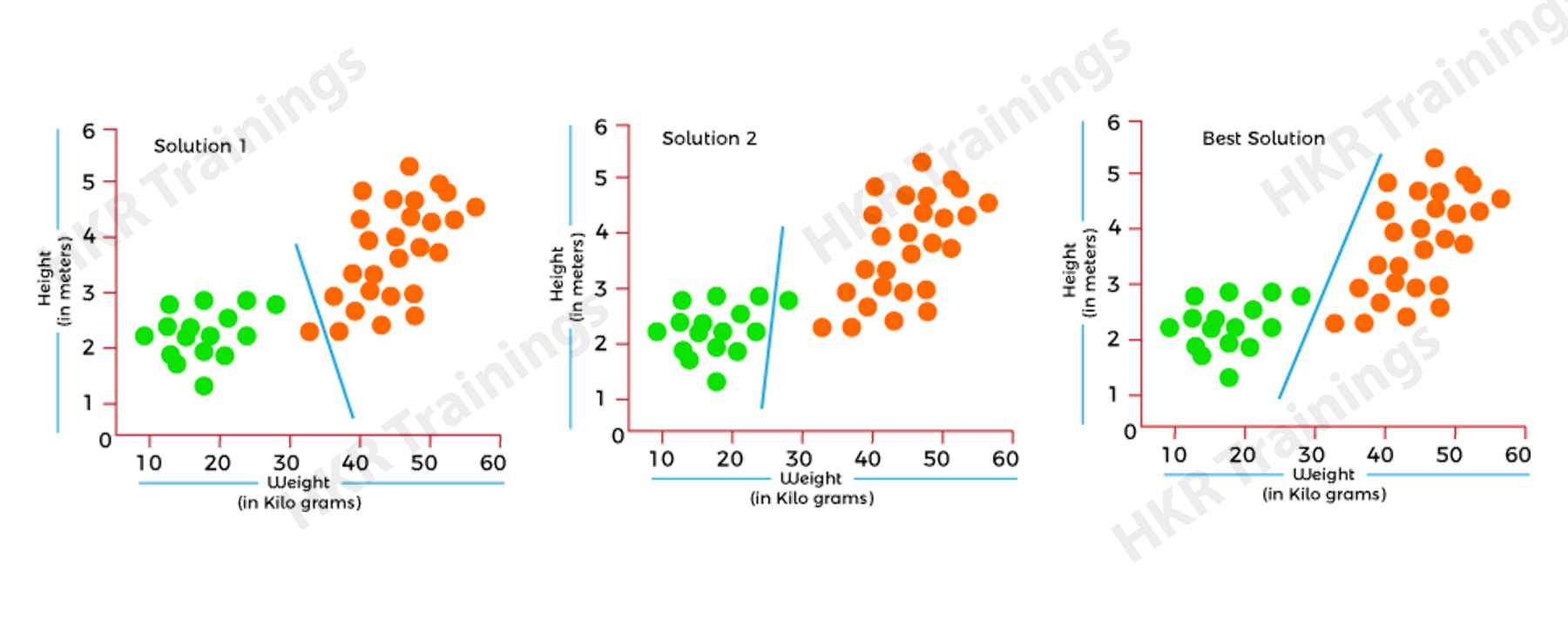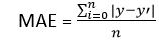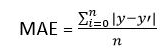# Cost Function in Machine Learning

A cost function is a very important parameter in the machine learning field which will determine the level of how good a machine learning model will perform with respect to the given dataset. It is essential to calculate the performance of a machine learning model once the user trains it. In this article, we will talk more about the use of cost function with the help of techniques in machine learning, the need to use cost function, the types of cost functions, and the need to minimize the cost function.

Cost Function in Machine Learning - Table of Content

## Cost Function

Cost function helps the user to calculate the performance of a model in this learning whenever the user trains it. There are several accuracy functions present as well that help the user understand how the model shows its performance, however, there are no suggested methods for improving the glitches. Hence, the user then requires a trained model which will specify the right point between the less trained as well as overtrained model. The cost function aims to give a real number as output by calculating the difference between the expected value to the predicted value.

We can also term the cost function as the Loss function. We can estimate it by performing an iterative run on the model for comparing the approximate predictions for the values of X and Y. The goal of each machine learning model is finding the value of parameters or their weights and can work on minimizing the parameters in that cost function.

### Use of Cost Function

Since we have a lot of accuracy parameters in machine learning, we still require the cost function model. Let us take an example and acknowledge it with the help of a data classification example below.

Let us assume that the user has a dataset consisting of the weights and heights of child 1 as well as child 2 and this dataset needs to be classified properly. The scatter plot that will form after plotting these 2 features is given below in image 1.In the image above, the orange dots represent child 1, and the green dots represent child 2. Now let us see and understand the best possible solution with the help of the classification method.As we can see in the image above, all the 3 classifiers have very high accuracy. However, if we look at the 3rd solution, it is the most appropriate and possible one as it is correctly classifying each data point. The right classification is defined as the mid-space between the two classes which is not much close and not even much far from each other.

We make use of the cost function to get these results.  It gives the most optimal solution as it calculates the difference b/w the original values and the predicted values.  It further measures the extent to which our model was wrong in predicting the same earlier. Hence, a user can obtain an optimal solution by reducing the cost function value.

Want to Become a Master in Machine Learning? Then visit here to Learn Machine Learning Training

### Machine Learning Training

• Lifetime LMS & Faculty Access
• Real-world & Project Based Learning

### Types of Cost Functions

The cost function can be of a number of types actually depends on the type of problem. But the most popular cost functions are categorized into 3 types which can be stated as:

#### 1. ‘Regression’ Cost Function

A user uses regression models for making predictions related to continuous variables like house prices, prediction of weather, prediction of loans, etc. When we make use of the cost function with Regression then it is termed a "Regression type Cost Function."

It can be calculated as an error that can be based on its distance by the formula below:

`Err= Actual Output - Predicted Output`

There are majorly 3 types of regression type cost functions. Let us have a look at them:

Means Error:

The user calculates the error for training and then calculates the mean for all the errors. This method is the easiest method.  The errors calculated from this method can be both negative as well as positive. They actually nullify each other giving the result as zero while finding the mean. Hence, it’s not much recommended for finding the cost function.

Mean Square Error (MSE):

This method is the most common method used for the Cost function. It aims at improving the drawbacks that come from mean error method. This method calculates the remainder between the original and the predicted value just like the mean error method but then squares it. As this method finds the double the difference in the values, it tends to avoid any chance of a negative error.

Below is the formula to calculate the mean square error of a function:This method is also called L2 loss. This is because each error is squared to avoid deviation while predicting the values. In case the dataset consists of more outliers, then this squaring art will multiply the errors and increase the inefficiency.

Mean Absolute Error (MAE):

This method also aims at overcoming the issue that comes with the mean abosulte error method. It takes up the actual difference b/w the actual and the predicted value. This method is also called L1 loss.  It is because this method does not get affected by any noise or outliers. Therefore it gives better results.

Below is the formula for calculating the mean absolute error of a function:If you want to Explore more about Machine? then read our updated article - Machine Tutorial !### 2. Cost Functions with  Binary Classification

The binary classification model is very useful for making predictions in the categorical variables like predicting for value zero or one, dog or cat, etc. We use cost function in the problem of classification and it is called the classification cost function. Though, this classification cost function is not similar to the Regression type cost function. We use a very popular loss function for the process of classification which is cross-entropy type loss.

Suppose the user wants to perform the process of classification between the colors blue and red. Hence, the binary classification process will come into play which is known to be an essential scenario in categorical cross-entropy. We only have one outcome class for that.

Below is the formula for calculating the mean absolute error of a function:

`Binary Cross Entropy = (Cross Sum - Entropy of X data) / X`

### 3. Cost Function as Multi-class Classification

This type of cost function that is very useful for classifying the issues related to instances that are subjected to more than 2 types of classes. This method is very similar to the binary classification cost function as cross-entropy and is also a common method for this type.

This method is designed to perform multi-class classification so that the user can achieve the goal values that range from zero to 1, 2 3, …. Upto n class. In this method, the categorical cross-entropy tends to generate a point that will summarise the basic mean difference b/w the actual and the probability distribution. It is assumed that to perform a great cross-entropy, 0 is the most ideal value and the score should always be minimized.

### How to minimize the cost function

This process of minimizing the cost function is just like coming down from a mountain. Suppose a climber is at the top of the mountain and he wants to descend. He would definitely want to opt for an easier path with the minimum number of steps from all the possible ways of coming down. There is a solution to this problem called gradient descent problem which can help in solving this.

Gradient Descent is actually a tool that helps in minimising the cost function. We can also say this tool helps in finding the minimum value of all the variables of the cost function. This process works in the direction that a user takes to reach the least possible error value. It is not necessary that the errors of the model are similar for different points, they can be different too. The aim is to find the easiest and quickest way to minimise it hence preventing resource wastage.

The user has to focus on the error of the model present for different input values. This method is repeated until the user finds that the value of error is getting smaller and smaller. The user will eventually arrive at a point where the error value will be the least and hence that way the cost function is optimised.

We understand that the error keeps decreasing constantly using gradient descent by its definition and hence reduces the cost function. This is done by calculating the difference between the errors. As small as the difference is, it can also be calculated by distinguishing the cost function and then performing subtraction on it from the last gradient descent in order to move down from the slope

### Machine Learning Training

Weekday / Weekend Batches

Conclusion

In this article, we have talked about the cost function in machine learning. A cost function is a very important parameter in the machine learning field which will determine the level of how good a machine learning model will perform with respect to the given dataset. We have also discussed the need to use cost functions, the types of cost functions, and the need to minimize the cost functions using the gradient descent method. The gradient descent method helps to minimise the error and hence reduces the cost function.

Related Article :

#### Find our upcoming Machine Learning Training Online Classes

• Batch starts on 5th Apr 2023, Weekday batch

• Batch starts on 9th Apr 2023, Weekend batch

• Batch starts on 13th Apr 2023, Weekday batch